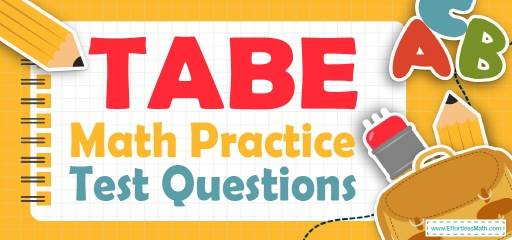# TABE Math Practice Test QuestionsPreparing for the TABE Math test? Try our free TABE Math Practice questions. Reviewing practice questions is the best way to brush up on your Math skills and measure your exam readiness. Here, we walk you through solving 10 common TABE Math practice problems covering the most important math concepts on the TABE Math test.

These TABE Math practice questions are designed to be similar to those found on the real TABE Math test. They will assess your level of preparation and will give you a better idea of what to study for your exam.

## 10 Sample TABE Math Practice Questions

1- $$5 \frac{1}{2}+8 \frac{1}{3}=$$

A. $$3 \frac{5}{6}$$

B. $$13 \frac{6}{5}$$

C. $$13 \frac{5}{6}$$

D. $$3 \frac{6}{5}$$

2- $$6 \frac{2}{5}-2 \frac{1}{5}=$$

A. $$4 \frac{1}{5}$$

B. $$3 \frac{1}{5}$$

C. $$7 \frac{1}{5}$$

D. $$12 \frac{1}{5}$$

3- $$5\frac{2}{3} -\frac{3}{4}=$$

A. $$5\frac{1}{12}$$

B. $$4\frac{1}{12}$$

C. $$4\frac{11}{12}$$

D. $$4\frac{6}{12}$$

4- $$\frac{2}{3} ×\frac{4}{5}=$$

A. $$\frac{2}{5}$$

B. $$\frac{4}{15}$$

C. $$\frac{8}{15}$$

D. $$\frac{4}{5}$$

5- $$\frac{4}{5}÷\frac{1}{10}=$$

A. $$\frac{4}{50}$$

B. $$\frac{5}{40}$$

C. $$\frac{1}{40}$$

D. $$8$$

6- $$2\frac{2}{3}×2 \frac{1}{6}=$$

A. $$2 \frac{7}{9}$$

B. $$5 \frac{9}{7}$$

C. $$5 \frac{7}{9}$$

D. $$2 \frac{9}{7}$$

7- $$12ab-5ab=$$

A. $$17ab$$

B. $$7ab$$

C. $$6ab$$

D. $$16ab$$

8- $$4^3×4^5=$$

A. $$4^{15}$$

B. $$4^{8}$$

C. $$4^{2}$$

D. $$4^{5}$$

9- $$4 \frac{3}{5} ÷2\frac{6}{10} =$$

A. $$1 \frac{10}{13}$$

B. $$1 \frac{13}{10}$$

C. $$\frac{10}{13}$$

D. $$2 \frac{13}{10}$$

10- $$5\%$$ of $$20=$$

A. $$5$$

B. $$4$$

C. $$3$$

D. $$1$$

## Best TABE Math Prep Resource for 2022

1- C
$$5 \frac{1}{2}+8 \frac{1}{3}=13 \frac{5}{6}$$

2- A
$$6 \frac{2}{5}-2 \frac{1}{5}= 6 + \frac{2}{5} -2-\frac{1}{5}=4 \frac{1}{5}$$

3- C
$$5\frac{2}{3} -\frac{3}{4}=4 \frac{11}{12}$$

4- C
$$\frac{2}{3} ×\frac{4}{5}=\frac{8}{15}$$

5- D
$$\frac{4}{5}÷\frac{1}{10}= 8$$

6- C
$$2\frac{2}{3}×2 \frac{1}{6}= \frac{8}{3} ×\frac{13}{6}=\frac{104}{18}=5 \frac{7}{9}$$

7- B
$$12ab – 5ab=7ab$$

8- B
$$4^3×4^5=4^{5+3}= 4^8$$

9- A
$$4 \frac{3}{5} ÷2\frac{6}{10} =\frac{23}{5} ÷\frac{13}{10}= \frac{115}{65}=1 \frac{10}{13}$$

10- D
$$5\%$$ of $$20 = 0.05 × 20 = 1$$

Looking for the best resource to help you succeed on the TABE Math test?

## More from Effortless Math for TABE Test …

### Do you need a full-length TABE math test to assess your math skills?

You are in the right place !: Full-Length TABE 11 & 12 Math Practice Test.

### Do you want to learn the math content of the TABE test in a few simple steps?

Use our Ultimate TABE Math Course (+ FREE Worksheets & Tests) for this purpose.

## Have any questions about the TABE Test?

### What people say about "TABE Math Practice Test Questions - Effortless Math: We Help Students Learn to LOVE Mathematics"?

No one replied yet.

X
52% OFF

Limited time only!

Save Over 52%

SAVE $40 It was$76.99 now it is \$36.99